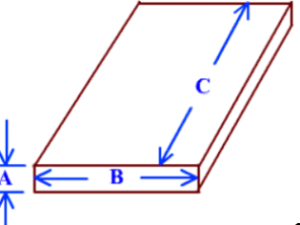## What is a board foot?

Q – Why do we need to know what a board foot of lumber is?

• You will be able to compare your final cost to a price from a lumber yard.
• You will know if you have enough logs to fill your lumber list/needs.
• I will be able to estimate how long it will take to mill your logs.
• You will be able to prioritize the cut list if you are short on logs.

Hardwood lumber that we use for framing, fencing, cabinetry and
furniture is sold by the board foot. This is a measurement that is
constant through all thicknesses of lumber, rather than applying to
“one-by twelve”, “two-by four”, etc.

###### See a Scribner Log Scale

Calculating Board Feet of Lumber

In the example piece of lumber below, the measurement “A” would be the thickness in inches , measurement “B” the width in inches, and measurement “C” the length in feet .Thus, the formula for calculating the board feet of lumber in our
example would be: ‘A’ times “B” times “C” divided by 12 or for example if the piece if lumber was
3 inches thick (A)
8 inches wide (B)
10 feet long (C), we would have
3 x 8 x 10 divided b12 = 20 board feet